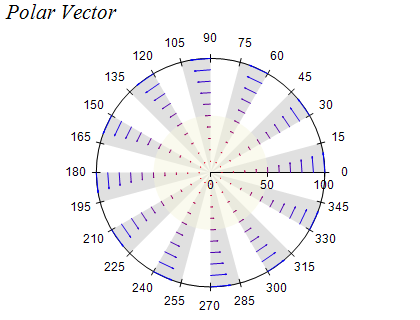﻿ Polar Vector
Nevron .NET Vision Documentation
Polar Vector

A Polar Vector chart displays vectors in a polar coordinate system. Each vector is specified by its begin and end points value and angle. The following figure displays a Polar Vector chart.Figure 1.

Creating a Polar Vector series

Polar Point series are represented by the NPolarPointSeries type. An instance of this type must be added to the series collection of a Polar Chart (NPolarChart object).

C#
Copy Code
```// clear the Charts collection and create a new polar chart
NPolarChart chart = new NPolarChart();
nChartControl1.Charts.Clear();
// add a polar vector series to the chart
NPolarVectorSeries polarVector = new NPolarVectorSeries();
```
Visual Basic
Copy Code
```' clear the Charts collection and create a new polar chart
Dim chart As New NPolarChart()
NChartControl1.Charts.Clear()
' add a polar vector series to the chart
Dim polarVector As New NPolarVectorSeries()
```
Passing Data

Once the series is created you can add some data in it. Polar vector series use the Values and Angles data series to store the vector begin coordinates and the Y2Values and X2Values to hold the vector end coordinates. The following code snippet adds a data point to a polar vector series:

C#
Copy Code

Visual Basic
Copy Code

Formatting commands

Besides the standard formatting commands you can use the following commands in format strings:

<angle_in_degrees> - the current data point begin angle in degrees (extracted from the Angles data series)
<angle_in_radians> - the current data point begin angle in radians (extracted from the Angles data series)

<angle2_in_degrees> - the current data point end angle in degrees (extracted from the X2Values data series)
<angle2_in_radians> - the current data point end angle in radians (extracted from the X2Values data series)

Related Examples
Windows forms: Chart Gallery\Polar\Polar Vector